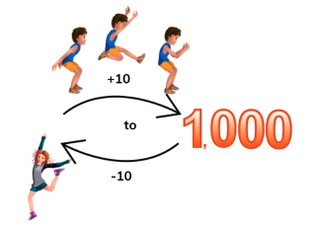Skip counting by 10s to 1,000

# Skip counting by 10s to 1,000

Skip count by 10s to 1,000

No account needed.8,000 schools use Gynzy92,000 teachers use Gynzy1,600,000 students use Gynzy

## General

Students learn to skip count forward and backward by 10s with numbers to 1,000.

## Standards

CCSS.Math.Content.2.NBT.A.2

## Learning objective

Students will be able to skip count forward and backward by tens to 1,000.

## Introduction

Show a numberline on the interactive whiteboard and say that you're going to make 4 jumps of 10. The first number is given. Have the students draw their own number lines and draw the correct number of jumps and to write which number they end up on. Then drag the arrows with +10 to the number line and say which number you end up on. Ask students to also make jumps of 10 backward on the number line.

## Instruction

Explain to students what tens are. Say that tens are a group of ten that end with a 0 in the ones place. It is important to know what tens are when you skip count by tens. Remind students that when you skip count by tens, the number in the ones place does not change, but the number in the tens place does. Show students a sequence in which you skip count by tens. Start at 600 and count on to 700. Point at the numbers and have the students count along out loud. Then show them a sequence starting at 408 and remind students that only the tens will change and not the ones. Show a number line in which the jumps of ten are shown. Ask students which numbers come next with jumps of ten. Have students write their answers on a sheet of paper or mini whiteboard and have them hold up their answers so you can check their work. Tell students that you can als skip count backwards by 10s. Point at the numbers and have students count backward with you by tens from 500 to 400. Then count together from 804 to 704. Remind students that again only the tens change when skip counting by tens, not the ones. Show the students a number line with a few question marks. Ask students which numbers belong at the question marks. As a class practice skip counting backward. Have students write the missing numbers on a sheet of paper or mini whiteboard and have them hold them up to check their work. Then have students drag numbers to where they belong on the given number lines.

Check that students are are able to count to 1,000 skip counting forward and backward by 10s by asking the following questions:
- What are tens?
- How do you skip count by tens?
- Skip count forward by 10s and begin at 573.
- Skip count backward by 10s and begin at 290.

## Quiz

Students are given tasks in which they skip count forward and backward first with support of a number line, and then asked to skip count without the visual support. Students are also asked to skip count forward and backward by tens.

## Closing

Discuss with students that it is important to be able to skip count by 10s to 1,000 so you can more easily count and build on math skills. To check that students understand, ask students to create the correct number sequences. They are given a starting number and must skip count forward by 10s. They must continue skip counting forward by 10s until there are no more numbers that fit the sequence.

## Teaching tips

Students who have difficulty with this learning goal can be supported by making use of the number line. Draw arrows so that students follow and know which direction they need to jump. Emphasize that when they skip count by 10s that only the number in the tens place changes, the ones stay the same. Start with practicing skip counting forward, and then move to skip counting backward.

### The online teaching platform for interactive whiteboards and displays in schools

• Save time building lessons

• Manage the classroom more efficiently

• Increase student engagement